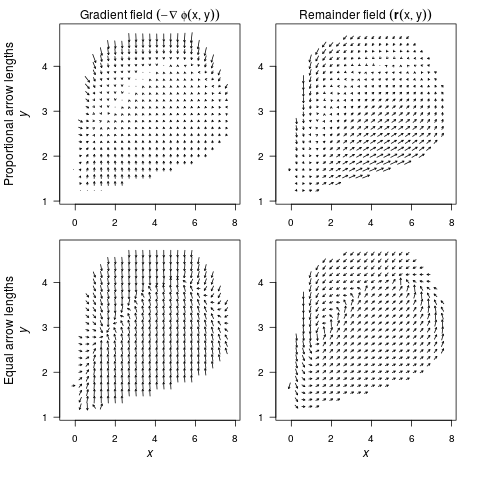In this vignette, we will extend the example from the R article and include the code to produce the figures.

### Example 1: A consumer-resource model with alternative stable states

``````    var.eqn.x <- "(alpha*x)*(1-(x/beta)) - ((delta*(x^2)*y)/(kappa+(x^2)))"
var.eqn.y <- "((gamma*(x^2)*y)/(kappa+(x^2))) - mu*(y^2)"
model.parms <- c(alpha = 1.54, beta = 10.14, delta = 1, gamma = 0.476, kappa = 1, mu = 0.112509)
parms.eqn.x <- Model2String(var.eqn.x, parms = model.parms)
parms.eqn.y <- Model2String(var.eqn.y, parms = model.parms)
``````

 “(1.54x)(1-(x/10.14)) - ((1*(x2)*y)/(1+(x2)))”

 “((0.476(x2)*y)/(1+(x2))) - 0.112509(y2)”

Here, we confirm that Model2String() correctly combined the equations and the parameters. We can suppress this output from Model2String() using suppress.print = TRUE.

### Step 1: Analyze the deterministic skeleton

To analyze our system of differential equations, we use the package phaseR, which requires the system of equations to be implemented as a function. Our function Model2String() was made to help users of QPot transition from their use of previous packages requiring this function format.

``````    require(phaseR)
model.ex1 <- function(t, y, parameters){
x <- y
y <- y
alpha <- parameters["alpha"]
beta <- parameters["beta"]
delta <- parameters["delta"]
kappa <- parameters["kappa"]
gamma <- parameters["gamma"]
mu <- parameters["mu"]
dx <- (alpha*x)*(1-(x/beta)) - ((delta*(x^2)*y)/(kappa + (x^2)))
dy <- ((gamma*(x^2)*y)/(kappa + (x^2))) - mu*(y^2)
list(c(dx,dy))
}
``````
``````    # draws the vector field
flowField(deriv = model.ex1, xlim = c(-0.1, 6), ylim = c(-0.1, 6), parameters = model.parms,
add = F, points = 30, col = "grey70", ann = F, arrow.head = 0.025, frac = 1.1,
xaxs = "i", yaxs = "i", las = 1)
# draw the nullclines and suppress the output by assigning it to a variable
supp.print <- nullclines(deriv = model.ex1, xlim = c(-0.1, 6), ylim = c(-0.1, 6),
parameters = model.parms, col = c("blue", "red"), points = 250)
# draw and label the equilibria
# open circles are unstable, black are stable
points(0,0 , pch = 21 , col = "black" , bg = "white" , cex = 1.5)
text(0,0 , labels = expression(italic(e[u1])) , adj = c(-0.1,-.25) , cex = 1.5)
points(1.4049, 2.8081 , pch = 21 , col = "black" , bg = "grey30" , cex = 1.5)
text(1.4049, 2.8081 , labels = expression(italic(e[s1])) , adj = c(-0.1,1.25) , cex = 1.5)
points(4.2008, 4.0039 , pch = 21 , col = "black" , bg = "white" , cex = 1.5)
text(4.2008, 4.0039 , labels = expression(italic(e[u2])) , adj = c(-0.1,1.25) , cex = 1.5)
points(4.9040, 4.0619 , pch = 21 , col = "black" , bg = "grey30" , cex = 1.5)
text(4.9040, 4.0619 , labels = expression(italic(e[s2])) , adj = c(.4,-.35) , cex = 1.5)
# label the x and y axis
mtext(expression(italic(x)), side = 1, line = 2.5)
mtext(expression(italic(y)), side = 2, line = 2.5)
``````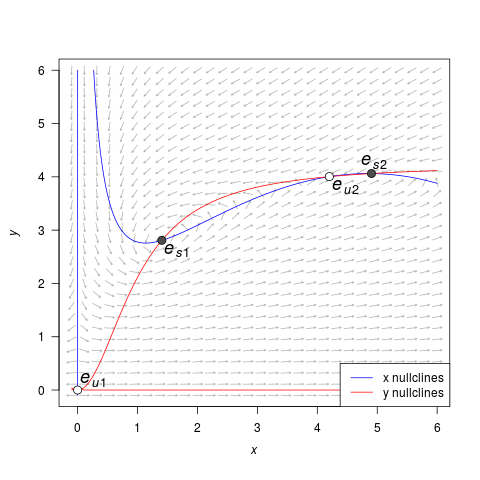### Step 2: Stochastic simulation

In order to simulate the stochastic equations, we need:

``````    model.state <- c(x = 1, y = 2)  # initial condition
model.sigma <- 0.05             # the level of noise
model.time <- 1000 #12500               # the length of the simulation
model.deltat <- 0.025           # the time step
``````

Then we can simulate the equations using TSTraj():

``````    ts.ex1 <- TSTraj(y0 = model.state, time = model.time,
deltat = model.deltat, x.rhs = var.eqn.x, y.rhs = var.eqn.y,
parms = model.parms, sigma = model.sigma)
``````

The function TSPlot allows us to easily view the output from TSTraj(). The default plot is the time series plotted through time with a histogram:

``````    TSPlot(ts.ex1, deltat = model.deltat)
``````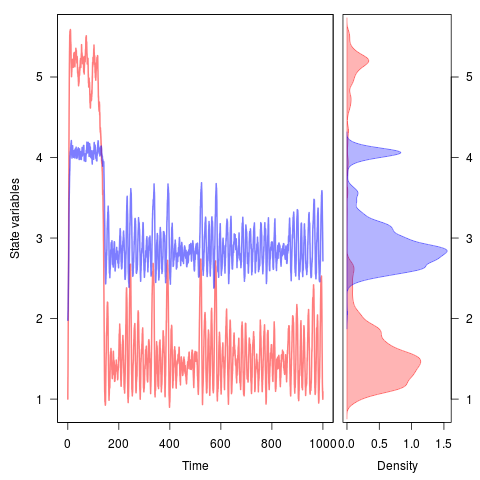We can view the times series plotted along on the state variables by setting dim = 2:

``````    TSPlot(ts.ex1, deltat = model.deltat, dim = 2)
``````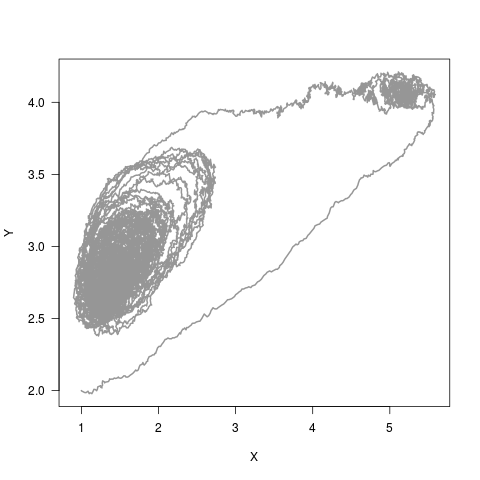If we only want to see the histograms, we can see them in one dimensional space

``````    TSDensity(ts.ex1, dim = 1)
``````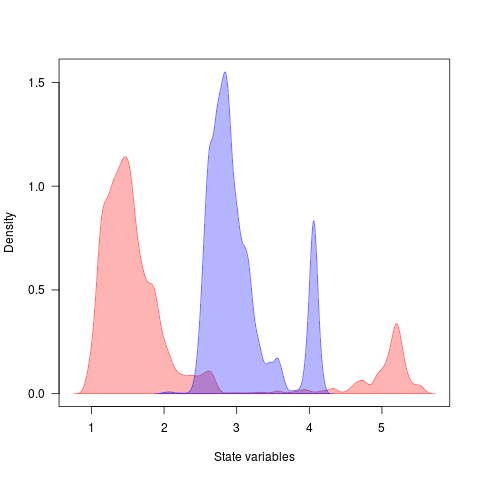or in two-dimensional space with

``````    TSDensity(ts.ex1, dim = 2)
``````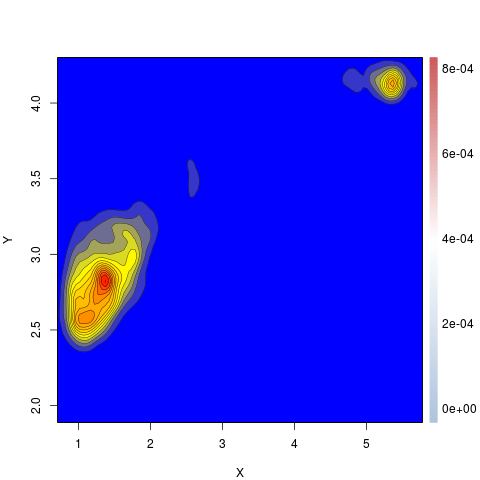### Step 3: Local quasi-potential calculations

To calculate the quasi-potential for a system of equations, we first need to define some initial conditions and parameter values.

``````    bounds.x = c(-0.5, 20.0)    # upper and lower limit of X
bounds.y = c(-0.5, 20.0)    # upper and lower limit of Y
step.number.x = 1000 #4100  # number of division between upper and lower limit
step.number.y = 1000 #4100  # number of division between upper and lower limit
eq1.x = 1.40491             # x value of first equilibrium
eq1.y = 2.80808             # y value of first equilibrium
eq2.x = 4.9040              # x value of second equilibrium
eq2.y = 4.06187             # y value of second equilibrium
``````

With these values defined, we can now compute the quasi-potential around each equilibrium point. Note that we don't have to start at the equilibria, but this ensures that we quickly find the lowest quasi-potential and work up from there.

``````    eq1.local <- QPotential(x.rhs = parms.eqn.x, x.start = eq1.x, x.bound = bounds.x,
x.num.steps = step.number.x, y.rhs = parms.eqn.y, y.start = eq1.y,
y.bound = bounds.y, y.num.steps = step.number.y)
eq2.local <- QPotential(x.rhs = parms.eqn.x, x.start = eq2.x, x.bound = bounds.x,
x.num.steps = step.number.x, y.rhs = parms.eqn.y, y.start = eq2.y,
y.bound = bounds.y, y.num.steps = step.number.y)
``````

The upwind ordered method will be chatty Set verboseC = FALSE to turn off completely Creating file name. File name created. Completed Memory Allocation equationx = (1.54x)(1-(x/10.14)) - ((1(x2)*y)/(1+(x2))) equationy = ((0.476(x2)*y)/(1+(x2))) - 0.112509(y2) hx = 0.0205205 hy = 0.0205205 Finished initializing a bunch of matrices in param() function cputime = 11.5584 Finished Loading Parameters Finished ipoint() function Initial count = 4 current count = 1000 51111 (2 101) is accepted, g=0.3012 Final count = 1228 Finished ordered_upwind() function cputime = 6.31942 Saves only to R In datasave case 2 Successful. Exiting C code The upwind ordered method will be chatty Set verboseC = FALSE to turn off completely Creating file name. File name created. Completed Memory Allocation equationx = (1.54*x)(1-(x/10.14)) - ((1(x2)*y)/(1+(x2))) equationy = ((0.476(x2)*y)/(1+(x2))) - 0.112509*(y2) hx = 0.0205205 hy = 0.0205205 Finished initializing a bunch of matrices in param() function cputime = 11.3052 Finished Loading Parameters Finished ipoint() function Initial count = 4 51113 (2 101) is accepted, g=0.2856 Final count = 1228 Finished ordered_upwind() function cputime = 7.11154 Saves only to R In datasave case 2 Successful. Exiting C code

We can use the function QPContour() to view the compute quasi-potential around each equilibrium. In some situations, this may be useful. For this example, it is not and we skip straight to computing the global quasi-potential and visualizing that.

### Step 4: Global quasi-potential calculation

QPGlobal() takes a list of local.surfaces (in this current example, two) and combines the output of QPotential() into a single global quasi-potential. We supply two points to help the function (in this case unstable equilibria) to tell QPGlobal() which points should have the same value. QPGlobal() uses these points to recalibrate the local quasi-potentials and combines these into a global quasi-potential.

``````    ex1.global <- QPGlobal(local.surfaces = list(eq1.local, eq2.local),
unstable.eq.x = c(0, 4.2008), unstable.eq.y = c(0, 4.0039),
x.bound = bounds.x, y.bound = bounds.y)
``````

### Step 5: Global quasi-potential visualization

``````        QPContour(surface = ex1.global, dens = c(1000, 1000), x.bound = bounds.x, y.bound = bounds.y, c.parm = 1)
QPContour(surface = ex1.global, dens = c(1000, 1000), x.bound = bounds.x, y.bound = bounds.y, c.parm = 5)
``````

The parameter c.parm modifies the distribution of contour lines throughout the quasi-potential (see the help file for specifics). But for right now, we can compare c.parm = 1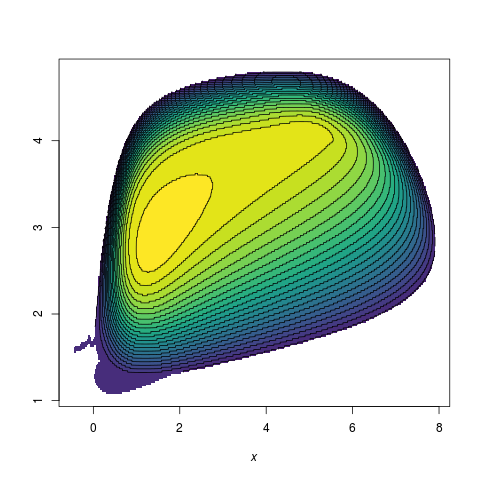with c.parm = 5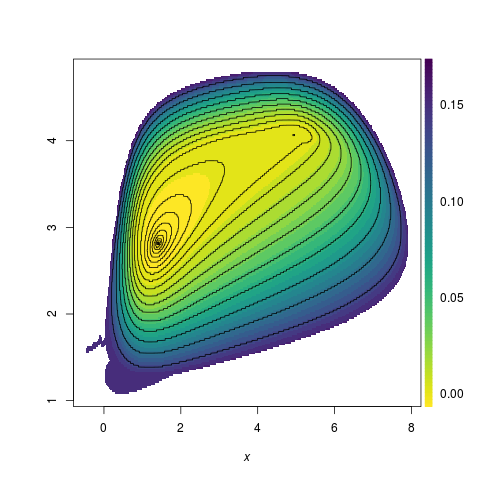In case you want to view the quasipotential in three dimensions, we can use the R package plot3D:

``````    require(plot3D)
require(viridis)
frac.x <- c(0.05, 0.3)
frac.y <- c(0.125, 0.25)
global.sub <- ex1.global[round(dim(ex1.global)*frac.x):round(dim(ex1.global)*frac.x),
round(dim(ex1.global)*frac.y):round(dim(ex1.global)*frac.y)]
global.sub[global.sub > 0.01] <- NA
#   par(fin = c(4, 4), oma = rep(0,4), mar = c(3.5, 3.5, 0, 0))
persp3D(z = global.sub, theta = 25, phi = 25, col = viridis(100, option = "C"), shade = 0.1,
colkey = list(side = 4, length = 0.85), contour = T, zlim = c(-0.001, .011),
zlab = intToUtf8(0x03A6))
``````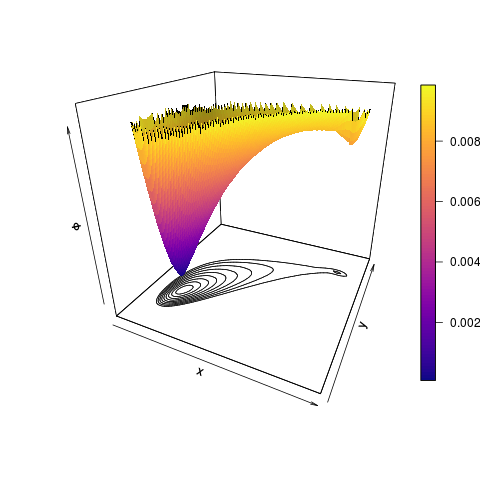### Step 6: Vector field decomposition

Taking the global quasi-potential, we can visualize the vector field, specifically the gradient and the remainder. First we calculate the vector field decomposition, which gives us the deterministic skeleton, the gradient vector field, and the remainder vector field for both state variables X and Y.

``````    VDAll <- VecDecomAll(surface = ex1.global, x.rhs = parms.eqn.x,
y.rhs = parms.eqn.y, x.bound = bounds.x, y.bound = bounds.y)
``````

We can then plot the deterministic skeleton

``````    VecDecomPlot(x.field = VDAll[,,1], y.field = VDAll[,,2], dens = c(25, 25),
x.bound = bounds.x, y.bound = bounds.y, xlim = c(0, 11), ylim = c(0, 6),
arrow.type = "equal", tail.length = 0.25, head.length = 0.025)
``````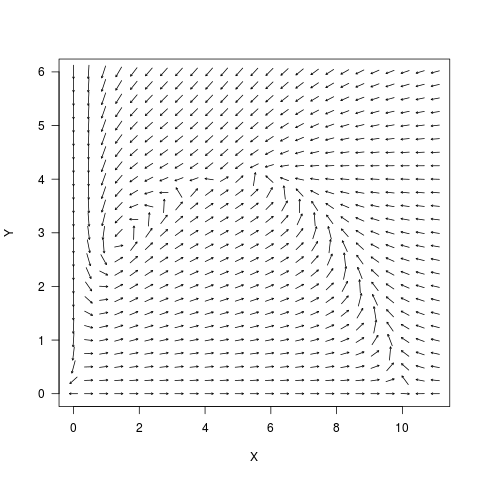followed by the gradient vector field

``````VecDecomPlot(x.field = VDAll[,,3], y.field = VDAll[,,4], dens = c(25, 25), x.bound = bounds.x,
y.bound = bounds.y, arrow.type = "proportional", tail.length = 0.25, head.length = 0.025)
``````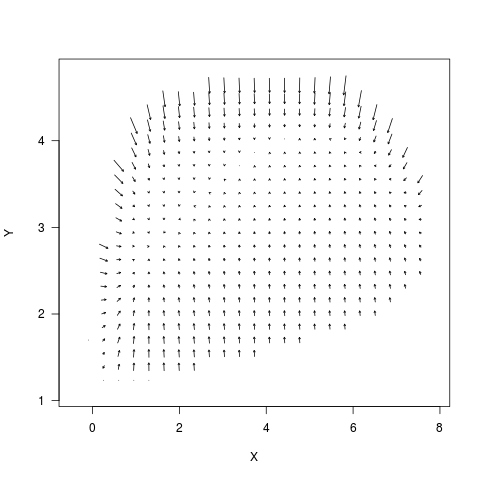and the remainder vector field, which is the “force that causes trajectories to circulate around level sets of the quasi-potential.”

``````VecDecomPlot(x.field = VDAll[,,5], y.field = VDAll[,,6], dens = c(25, 25), x.bound = bounds.x,
y.bound = bounds.y, arrow.type = "proportional", tail.length = 0.35, head.length = 0.025)
``````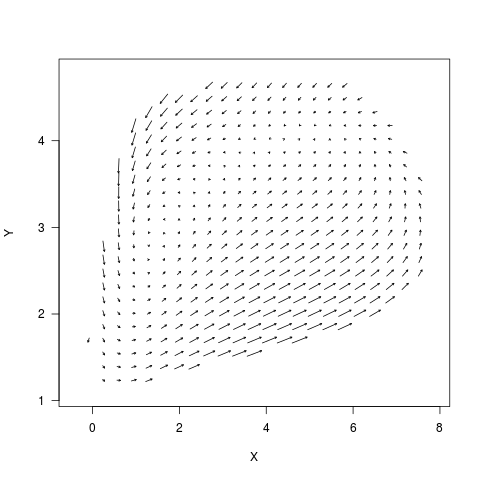The force arrows can be drawn equal size or can be drawn proportional to their value by setting arrow.type to “equal” or “proportional”.